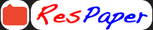Trending ▼   ResFinder# ICSE Class X Prelims 2021 : Physics (St. Mary's School ICSE, Mazagaon, Mumbai)

2 pages, 18 questions, 0 questions with responses, 0 total responses,20Aashir Moh.d J.S.S. International School (JSS IS), Dubai
+Fave Message
 Home > aashirhd >   F Also featured on: School Page icseFormatting page ...

St. Mary s School (I.C.S.E), Mazagaon (1st Term Assessment, November 2020) Physics Date :09/11/2020 Class : 10 Total Marks : 40 Total Time 1 hr. Section (A) :20 Marks Q.1.) Define moment of force and state its S.I. unit.  Q.2.) The moment of a force of 5N about a point P is 2Nm. Calculate the distance of point of application of the force from the point P.  Q.3.) Define centre of gravity.  Q.4)  State the position of centre of gravity for (i) hollow cylinder (ii) hollow cone. Q.5.) Calculate the power of a machine required to lift 105kg of stone per hour from a depth of 360m. (g = 10 m/s2)  Q.6.) State the energy conversion for (i) lighted electric bulb (ii) petrol engine of a car.  Q.7.) State two characteristics of a fuse.  Q.8.) How does the resistance of a wire depend upon its (i) length and (ii) area of cross section ? Q.9.) The refractive under of water is respected to water? 4 3 and of glass is . What is the refractive index of glass with 3 2  Q.10.) Draw a neat labeled diagram of apparent bending of a stick under water.  Section (B): 20 Marks Q.11.) (a) A ball of mass 20g falls from a height of 10m and rebounds from the ground to 4m. Find (i) Initial P.E. of the ball (ii) K.E. of the ball just before striking the ground (iii) Loss in K.E. of the ball on striking the ground (g = 9.8 m/s2). Why is there a loss in K.E.?  Continued on the next page .Formatting page ...